## NCERT Solutions for Class 9 Science Chapter 16 Floatation

These Solutions are part of NCERT Solutions for Class 9 Science. Here we have given NCERT Solutions for Class 9 Science Chapter 16 Floatation

More Resources

NCERT TEXT BOOK QUESTIONS

IN TEXT QUESTIONS

Question 1.
Why is it difficult to hold a school bag having a strap made of a thin and strong string ?
It is painful to hold a heavy bag having strap made of a strong and thin string.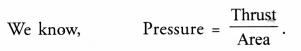When we hold a heavy bag having strap made of a strong and thin string, then the area under the strap is small. Hence, large pressure is exerted by the strap on our fingers or shoulder. Due to this large pressure, the strap tends to cut the skin and hence pain is caused.

Question 2.
What do you mean by buoyancy ? (CBSE 2011, 2012, 2016, 2017)
The tendency of an object to float in a liquid or the power of liquid to make an object to float in it is called buoyancy.

Question 3.
Why does an object float or sink when placed on the surface of water ? (CBSE 2012)
When an object is placed on the surface of water, two forces act on the object.

1. The gravitational force in the downward direction and
2. Upthrust of the water on the object.

When the gravitational force acting on the object is greater than the upthrust of the water, then the object sinks in water. On the other hand, if the upthrust of water on the object is greater than the gravitational force, then the object floats.

Or

If density of an object is less than the density of water, it floats on the water. On the other hand, if density of the object is greater than the density of water, it sinks in water.

Question 4.
You have a bag of cotton and an iron bar, each indicating a mass of 100 kg when measured on a weighing machine. In reality, one is heavier than other. Can you say which one is heavier and why ?
(CBSE 2011)
Iron bar is heavier than a bag of cotton. This is because iron bar experiences a less upward force i.e. upthrust than the bag of cotton.

NCERT CHAPTER END EXERCISE

Question 1.
In what direction does the buoyant force on an object immersed in a liquid act ?
Buoyant force acts in the upward direction on an object immersed in a liquid.

Question 2.
Why does a block of plastic released under water come upto the surface of water ?(CBSE 2011, 2016)
This is because upthrust or buoyant force acting on the block of plastic is greater than the gravitational force acting on the block in the downward direction.

Question 3.
The volume of 50 g of a substance is 20 cm3. If the density of water is 1 g cm-3, will the substance float or sink ? Justify your answer. (CBSE 2011, 2012)Since density of substance is more than the density of water, so the substance will sink.

Question 4.
The volume of a 500 g sealed packet is 350 cm3. Will the packet float or sink in water if density of water is 1 g cm-3 ? What wall be the mass of the water displaced by the packet ?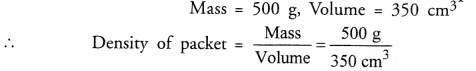Since the density of the packet is greater than the density of water, so the packet will sink.
Mass of water displaced= Volume of Packet x density of water = 350 x 1 = 350 g.

PRACTICAL SKILL BASED QUESTIONS

Question 1.
Write two main precautions to be taken to read the water level in the graduated cylinder. (CBSE 2015)

1. The cylinder must be placed on a flat surface in exactly vertical positoin.
2. The level of eyes must be exactly at the level of lower end of the meniscus of water.

Question 2.
Write precuations to be taken to measure the weight of a body using a spring balance. (CBSE 2015)

1. The least count of the spring balance must be calculated.
2. Zero error of the spring balance must be calculated and added or substracted from the observed value of the . weight of the body.
3. The level of eyes must be exactly at the level of the pointer of the spring balance.

Question 3.
A 500 g mass body is immersed in two liquids X and Y in succession. The extent to which the body sinks in liquid Y is less than that in liquid X. From such observation, compare the densities of liquids X and Y. Justify your answer. (CBSE 2015)
Weight of body immersed in liquid = Weight of body in air -upthrust of liquid on the body.
Since the extent to which the body sinks in liquid Y is less than that in liquid X, therefore, upthrust of liquid Y is greater than the upthrust of liquid X.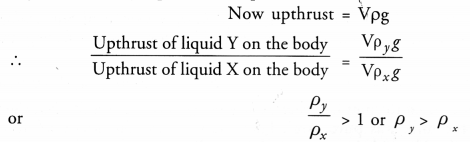Thus, density of liquid Y is greater than the density of liquid X.

Question 4.
In a spring balance, the space between 0 to 25 g wt. is divided into 5 equal parts. Find its least count. What is the weight of the object in the diagram given below ? (CBSE 2015)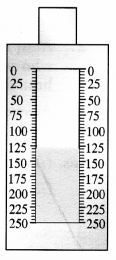5 divisions = 25 gwt
1 divisions = 5 gwt
Hence, least count of the spring balance = 5 gwt.
Weight of object in the diagram = 125 x 5 gwt = 625 g wt

Question 5.
While determining the density of a solid of weight 58 g wt, a student is provided with four different combinations of mesuring cylinder and spring balance as shown below :
The student should prefer to choose which combination and why ? (CBSE 2015)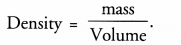Density can be determined accurately if mass and volume of solid are measured accurately. Therefore, mass must be determined by a spring balance of minimum least count and volume must be determined by a measuring cylinder of minimum least count. Hence, student should prefer the combination 1.

Question 6.
Observe the two spring balances shown in the figures given aside :

1. What are the least count and range of the spring balances ?
2. Find the weights of objects in the two spring balances. (CBSE 2015)1. In both spring balances, 5 divisions = 10 kg
1 division = 2 kg
Hence, least count of both spring balances = 2kg.
Range of both spring balances = 0-50 kg
2. Weight of object measured by spring balance A = 10 kg
Weight of object measured by spring balance B = 40 kg
A spring balance used for measuring mass of the cuboid has a least count of 2 gwt.

Question 7.
Two students using the same spring balance noted two different readings, 46 gwt and 47 gwt. Which reading is correct and why ? (CBSE 2015)
The reading which is the multiple of least count (i.e. 2 gwt) is correct. Therefore, 46 gwt is the correct reading.

Question 8.
A 10 mL measuring cylinder has 100 divisions of equal spacing. Find the least count of the measuring cylinder.
Least count of measuring cylinder = Value of 1 division marked on the cylinder 100 divisions =10 mL .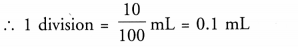Therefore, least count of the measuring cylinder = 0.1 mL

Question 9.
In which of the two, glycerine or kerosene, the loss in weight of a solid when fully immersed in them will be more and why ? (CBSE 2017)